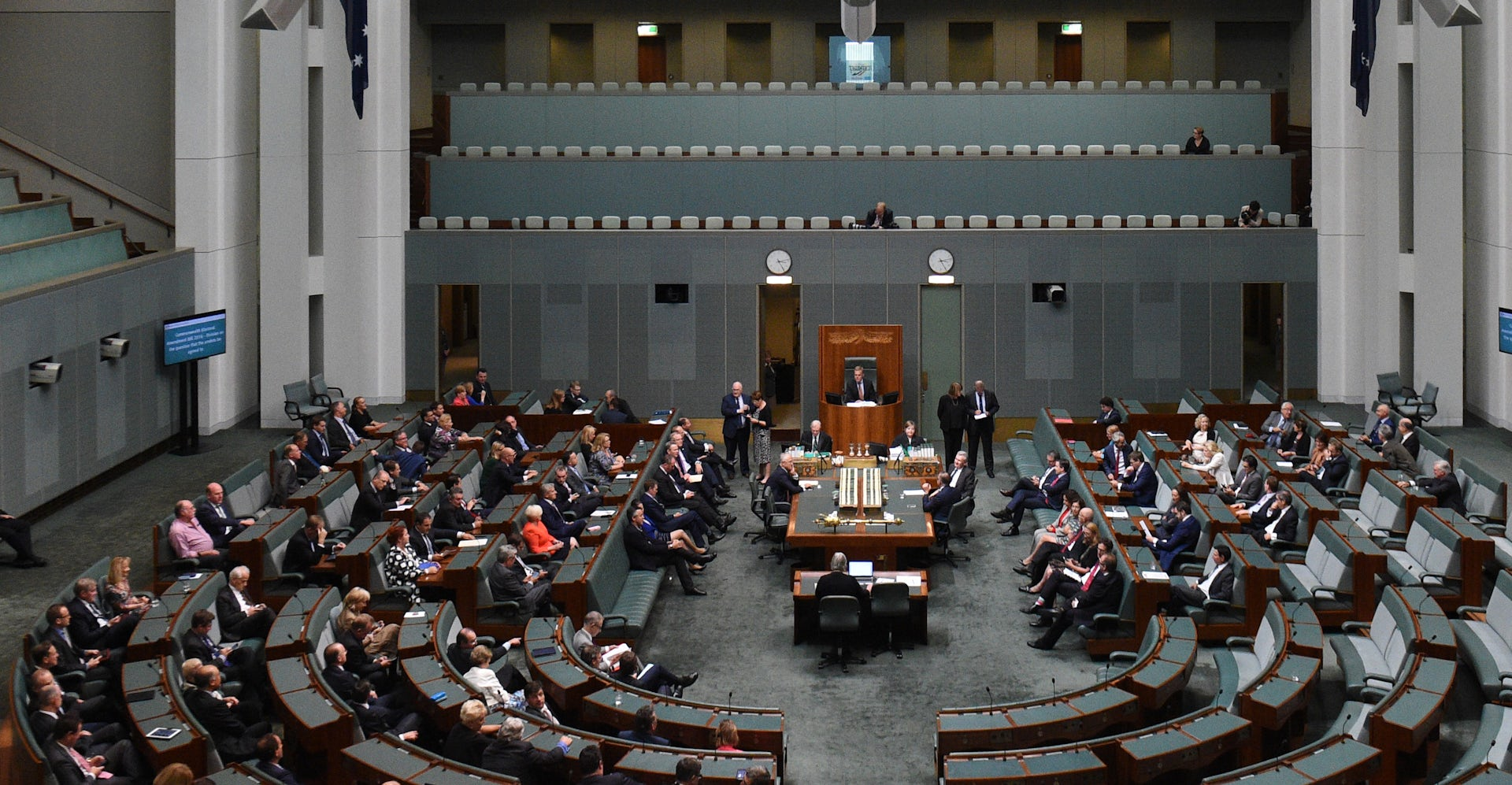Why does the number of members of Australia’s lower house fluctuate? AAP/Mick Tsikas

When Australians go to the polls on July 2, they will elect 150 members of the House of Representatives. But, in 1998, there were 148 lower house MPs. So why does this number fluctuate? And what are the lowest and highest possible numbers?

## How to work it out

There are 435 members of the US House of Representatives and 650 members of the UK House of Commons. These numbers are set by legislation and can be changed by a vote.

In Australia, however, the number is variable. It is a result of a formula given in the Constitution. Three principles form the basis of determining the number:

• there are 76 senators: 12 from each of the six states and two from each of the two territories;

• as per Section 24 of the Constitution, the number of members of the House should be “as nearly as practicable” twice the number of state senators (72): so, close to 144; and

• the House is comprised via popular representation. The more populous a state, the more members it should have.

From these three principles emerges a formula. Let P be the population of New South Wales, say, and let C be the population of Australia. The number of members representing NSW should be “roughly” P divided by C, then multiplied by 144.

In 2014, the Australian Electoral Commission determined the number of seats in the House of Representatives ahead of the 2016 election. NSW’s population was 7,500,617; Australia’s was 22,793,303. Therefore, the number of members in NSW should be roughly 7,500,617 divided by 22,793,303, then multiplied by 144. This is about 47.3862.

Now we calculate the number of members of each of the states and territories and total them. We have (P1/C) x 144 + (P2/C) x 144 + … + (P8/C) x 144, where the P1, P2 and so on are the populations of the eight states and territories.

Since 144/C is a common term in the sum, we can factor this out, leaving 144/C (P1 + P2 + … + P8). Since the sum of all of the populations is C, we have 144 x C/C = 144. Thus, “roughly” the number of members will be 144, which is twice the number of the state senators.

But, we have swept a lot under the rug by using the term “roughly”.

First, the number of seats needs to be a whole number. There can’t be 47.3862 members for NSW. So, if (P/C) x 144 has a remainder less than or equal to half it is rounded down. Otherwise, it is rounded up.

Second, no state can have fewer than five seats and no territory can have fewer than one.

So, if we apply these changes and use the population statistics from the Australian Bureau of Statistics, we arrive at the current composition of 150 members.

## Small houses and large houses

Can we think of a scenario in which the number of members was maximised or minimised?

Consider first the minimum number of members. Note that the number (P/C) x 144 can be rounded down only if the decimal part does not exceed one-half.

If, say, NSW’s allotment came out as (P/C) x 144 = 48.5, then NSW would be awarded only 48 seats. If the decimal part for each of the states and territories was one-half, then the total number of seats to be awarded would be (P1/C) x 144 minus one-half + (P2/C) x 144 minus one-half + … (P8/C) x 144 minus one-half. This is equal to 144 minus four, or 140.

Now consider the maximum number of members. Suppose, in a simplified model, that there were only two jurisdictions: NSW and the ACT, and that NSW had 99% of Australia’s population. A little algebra shows that NSW would have 143 seats and the ACT one: a total of 144 members.

Now suppose that almost everyone moved to Canberra, and that NSW only had 1% of the population. The ACT boosts its total to 143. NSW only “deserves” one seat, but, since it is an initial state, it must have at least five. This gives a total of 148 members.

This shows us how to maximise the number of members. Suppose that all the states were sparsely populated, owing to a mass emigration to the territories. The states “transfer” their seats to the territories, but pick up the consolation prizes of a minimum of five seats each. This yields an almighty 175-member lower house.

### Want to write?

Write an article and join a growing community of more than 89,700 academics and researchers from 2,958 institutions.